Journal of Finance and Accounting
Volume 3, Issue 5, September 2015, Pages: 103-116

Analysis for Efficient Stock Index Market1

Byung Woo Kim

Division of Liberal Arts, Korea National University of Transportation(KNUT), ChungJu City, South KoreaByung Woo Kim. Analysis for Efficient Stock Index Market. Journal of Finance and Accounting. Vol. 3, No. 5, 2015, pp. 103-116. doi: 10.11648/j.jfa.20150305.11

Abstract: According to efficient market hypothesis, all the available information about future spot prices is incorporated into KOSPI200 stock index future contract prices immediately. In this paper, we test the hypothesis by an econometric approach developed by Johansen (2001), etc. Using only past data, we can consider stock market as efficient. (Weak-form EMH) But, it should be noted that if using past index future contract data leads to conclusion that future market is not efficient, and then the news about spot market prices is not fully incorporated in the future market. These tests imply that unbiasedness hypothesis (constant is zero, and coefficient is one) is rejected in regression equation. Notwithstanding, the forecasting performance of the Distributed-lags model using future index was best among competing forecasting models. In summary, there is no significant evidence that stock index spot markets are inefficient. Through long-run equilibrium relationships (and short-run dynamics, error correction), almost all the information for spot index in the past has functioned as spot price discovery in Korea stock markets.

Keywords: Efficient Market, Nonstationarity, Price Discovery, Conditional ECM, Cointegration

1. Introduction

In Econometrics, the efficiencies of spot and future stock index markets are tested. Tests for the getting opportunity of excess returns are also performed. Efficiency means that the expected value of excess rate of return in stock index is zero.

And, if future market is efficient, future price index can be good predictor for spot index. In an informational efficient asset markets, price changes should be unforecastable if market fully incorporates the expectations. The predictability of asset returns is closely related with beating the market or market efficiency.

Fama (1970) provides the idea that efficient market always fully reflects available information. Fama (1998) provides the empirical event studies

Focusing on long-run return anomalies of reactions to information. Yen and Lee (2008) survey articles that the Efficient Market Hypothesis (EMH) no longer gets support from scholars. The emergence of behavioral finance in the 1990s, contributed to this environment.

Schulmeister (1987) presents that there are some limitations of stock market efficiency by providing evidence of the possibility of excess returns through transaction strategy in foreign exchange market. Levich (2001) tests for the existence of excess returns using filter rule and moving average crossover rule.

Engle (1982)’s original work on ARCH was about the volatility of inflation. But, it was the analysis of financial time series that consolidated the importance of his contribution. Campbell, Lo and MacKinlay (1997) introduce Financial Econometrics that tests market efficiency empirically. They reject random walk hypothesis 3(RW3) over sub-sample periods using CRSP equal and value weighted market return indices.2

Lim and Brooks (2011) provide a review of the weak-form market efficiency literature that examines predictability from past data. The result is that the evidence of stock return predictability can be justified within the adaptive markets hypothesis.

Bae, Kwon and Park (2004) surveys previous literatures and performed empirical tests for market operational efficiency. In Korea, index futures trading on the Korea Stock Exchange (KSE) was introduced in May 1996.

In this study, we examine the problem of predicting future price change, using only past price change and past future price changes. We use unit root test for assessing market efficiency of KOSPI index. This may be an alternative remedy for OLS estimator that has some defects with statistical inference.

Figure 1. depicts the trends in the spot and future stock index in the Korean security markets.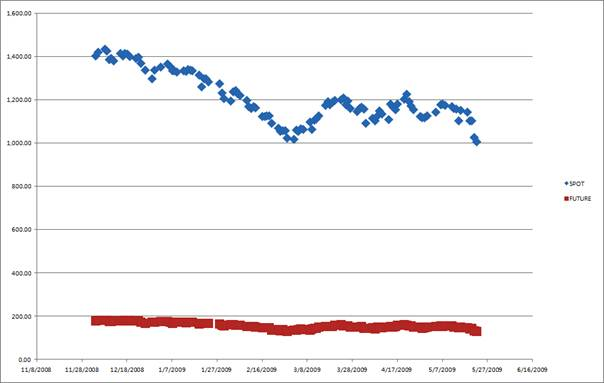Figure 1. Trend of Stock Index (Source: Korea Stock Exchange [KSE]).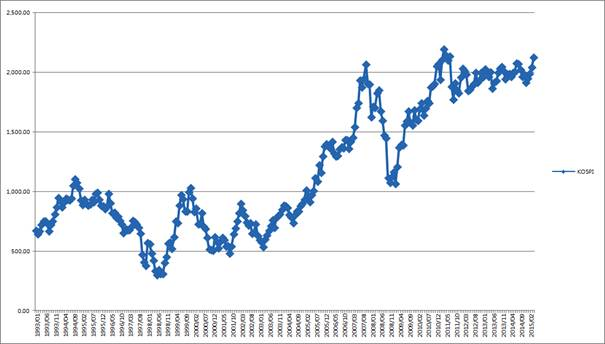Figure 2. Trend in Korea Stock Price Index-KOSPI (Source: BOK).

The underlying asset in Stock Index Futures is Stock Index and not individual equity. The settlement process is specific in that a special settlement index is used in cash liquidation. If future market is efficient, future prices can have high forecasting power for spot prices. This means that future price incorporates much information in markets with regard to future spot price.

It seems to be that almost all the available information about future spot prices is incorporated into current and past spot prices immediately. In this paper, we test the hypothesis by using past data and concluded that unbiasedness hypothesis (constant is zero, and coefficient is one) is not rejected in random walk equation. If using only past data, we can consider stock market as efficient. (Weak Form EMH)3

But, it should be noted that if using past index future contract data leads to the conclusion that future market is not efficient, and then the news about spot market prices is not fully incorporated in the future market. The cause of these inefficiencies needs to be examined further in future research.

In summary, there is no significant evidence that stock markets are inefficient. Through long-run equilibrium relationships (and short-run dynamics, error correction), all the information for forthcoming spot index has been incorporated as spot price discovery in future in Korea stock markets.

In this paper, we review traditional unbiased hypothesis with KOSPI200 Future Index. We deal with the issue of efficient market hypothesis. We examine the proposition that the best predictor for future spot price index is the future price. In addition, we adapt simple econometric model to recent Korean market data by empirical analysis. Our strategy is as follows: first, we test random walk hypothesis by AR estimation and unit-root test. Then, we examine the efficiency of index future market by least squares(distributed–lags), VAR and cointegration estimation. Finally, we conclude the predictability of stock index prices in both spot and future market by overall examination of empirical results.

2. Economic Model and Empirical Analysis

2.1. Data

The data set consists of spot index of KOSPI and future index (KOSPI 200 F 201506) observed for 240 days (2014:6:13-2015:5:21) in Korea. They were obtained from BOK, KOSIS and KRX (Korea Exchange).

Stock index futures on the Korea Composite Stock Index (KOSPI) 200 were introduced in May 3, 1996 by the KSE (Korea Stock Exchange). KSE had published the KOSPI 200 list on June 15, 1994. Mainly traded asset in future market is stock index futures. Rates of return were obtained by log difference. (SDIFF for spot index, FDIFF for future index, respectively)

Table 1. Summary Statistic for Variables (240 days, 2014:6:13-2015:5:21).

 KOSPI KOSPI200 (Futures) Mean 2004.09 257.38 SD 64.52 8.10 Sample 240 231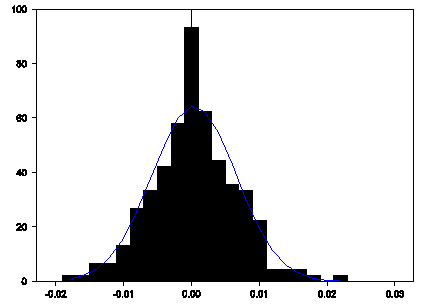Figure 3. Histogram of the Spot Returns.

Fig 3. shows the histogram of the returns. It displays non-normal properties. There are more observations around the mean and in the tails. These are said to be leptokurtic.

2.2. Efficient Market Hypothesis

Weak-form market efficiency argues that the information includes only historical prices (returns) themselves. The earliest model of asset prices is the martingale model. This contains the notion of fair game which could be found in contingent commodity model. Fair game is one for which the expected price next period is equal to this period’s price, conditional on the history. If stock market is efficient, investors cannot get excess rate of return. It states that the expected value of rate of return is zero.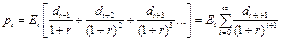(1)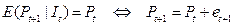(I: available information at t)

Current stock price is (conditional) expected present value of some of future dividends.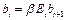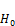(2)

In general, the effects of efficiency is expressed that current price is best predictor for future stock price. This is tested in simple regression whether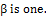(equation 2)

2.3. Random Walk and Unit Root Tests for Stationarity

In general, the effects of temporary macroeconomic policy are transitory according to the traditional Keynesian model. But, if output series has unit root, the effects are permanent. The same logic may be applied to the stock price index series.

Consider the AR (1) model with constant and white noise innovations.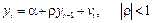(3): α=0, ρ=1

First, we used OLS estimator for determining market efficiency of spot series. The random walk 1(RW1) hypothesis argues that constant and coefficient are zero and one respectively.5 Wald test for coefficient restrictions reveals that random walk (non-predictability of index level) is well fitted to data.

Before we perform unit root test for stock price index series, we used OLS estimator for determining the stationarity of series. In this case, conventional t table is inappropriate. So, unit root test in AR equation is appropriate. (Equation 3) The random walk 1(RW1) hypothesis argue that error is independently and identically distributed (IID). This may be tested also by traditional statistical tests (Kendall Tau correlation test etc.) and Runs test (Cowles-Jones ratio). The random walk 2 (RW2) hypotheses argue that errors are independent increments. This may also be tested by filter rules and technical analysis. These tests may be postponed to future research.

Table 2. AR (1) Model for Stock Price Index.

 LOG(S) Variable Coeff Std Error T-Stat Signif Constant 0.06 0.09 0.63 0.52 LOG(S){1} 0.99 0.01 76.48 0.00**
 Null Hypothesis: C(1)=0, C(2)=1 Null Hypothesis Summary: Normalized Restriction (= 0) Value Std. Err. C(1) 0.087180 0.096360 -1 + C(2) -0.011431 0.012675 Restrictions are linear in coefficients.

Now, we use critical values for the Dickey-Fuller (1981) test, instead, Augmented Dickey-Fuller unit root test allows autocorrelation of error term.(4)

In financial econometrics, the persistence of stock index has sparked many considerations. Thus to test for trend reversion vs. permanent shocks, we test the null hypothesis that γ=0. This tests whether index does not revert toward a fixed mean. Formally, it means that the series has a unit root.

Meanwhile, we should not confuse test of Random Walk with that of unit root. Unit root test is not for the predictability but permanent/transitory nature of shocks to asset returns (prices).

Table 3. Dickey-Fuller Unit Root Test.

 Dickey-Fuller Unit Root Test, Series LOGKOSPI T-test statistic -0.82 Dickey-Fuller Unit Root Test, Series LOGKOSPIF T-test statistic -1.22 Critical values: 1%= -3.46 5%= -2.87 10%= -2.57

Campbell, Lo and MacKinlay (1997) introduce the methods for tests of Random Walk 3(RW3, uncorrelated increments). Romano and Thombs (1996) derived asymptotic approximations which may be used to construct tests of random walk. Q-statistics from the residuals in the AR (1) regression due to Box and Pierce (1970) are not significant at all lags, indicating no significant serial correlation in the residuals. Breusch-Godfrey Serial Correlation Lagrange Multiplier test also shows market efficiency indirectly.

One method for using long-horizon returns is the permanent/transitory components, proposed by Muth (1960). Variance ratio, which is (signal/signal+noise) ratio, tests for the hypothesis that series is a random walk 3(RW3). Our test results reject this proposition at high significance. This is unique evidence that market is inefficient in this study. In later section, we provide some explanations for inference results of inefficient market. In general, this test is used in analyzing economic variables such as dividend-price ratio. (Campbell et al. 1997)

The random walk 3 (RW3) hypotheses argue that errors are uncorrelated increments. This may also be tested by autocorrelation coefficients, Portmanteau statistics and variance ratio tests.

Table 4. Autocorrelation, LM and Variance Ratio Tests For Market Efficiency (RW3).

 AC PAC Q-Stat Prob 1 0.022 0.022 0.1128 0.737 2 0.036 0.035 0.4149 0.813 3 -0.002 -0.004 0.4163 0.937 4 -0.002 -0.003 0.4173 0.981 5 0.008 0.008 0.4330 0.994 6 -0.068 -0.068 1.5348 0.957 7 -0.004 -0.002 1.5391 0.981 8 0.021 0.026 1.6417 0.990 9 -0.043 -0.044 2.0791 0.990 10 -0.058 -0.059 2.8993 0.984 11 0.080 0.088 4.4656 0.954 12 0.074 0.071 5.7991 0.926
 LM Test: F-statistic 0.309263 Prob. F(2,225) 0.7343 Obs*R-squared 0.627797 Prob. Chi-Square(2) 0.7306 Null Hypothesis: SDIFF is a martingale Joint Tests Value df Probability Max |z| (at period 2)* 6.032347 229 0.0000 Individual Tests Period Var. Ratio Std. Error z-Statistic Probability 2 0.494723 0.083761 -6.032347 0.0000 4 0.260991 0.145717 -5.071537 0.0000 8 0.129149 0.212186 -4.104188 0.0000 16 0.073542 0.302376 -3.063923 0.0022 *Probability approximation using studentized maximum modulus with parameter value 4 and infinite degrees of freedom

Almost all the previous studies show the evidences that market is efficient, but conclusion is mixed. Focusing on the opportunity of getting excess returns, researches mostly use filter rule and moving average crossover rule methods.(Levich 2001)9 But, we should note that excess returns are not necessarily imply market inefficiency, since they incorporate part of risk premium.

3. Efficiency in Future Market

3.1. Spot and Future Price Index Data

We use data set that consist of spot index of KOSPI and future index (KOSPI 200F) for examining efficient market hypothesis.

If stock market is efficient, then future price is best predictor for spot prices in the future.

F = E ((5)

This argument is expressed by the hypothesis test problem.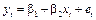(x=Lagged Future, y=Spot):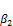=1,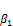=0

The empirical tests for the efficiency of future market are focused on whether future returns (or variables) can forecast the future spot returns or not. This idea is based on the inference that if market is efficient, then future market can incorporate information about future spot prices well. But, previous literatures provide negative answers for this hypothesis. Bae et al. (2004) test this proposition by setting simple actual (security) price adjustment model of Brorsen et al. (1989)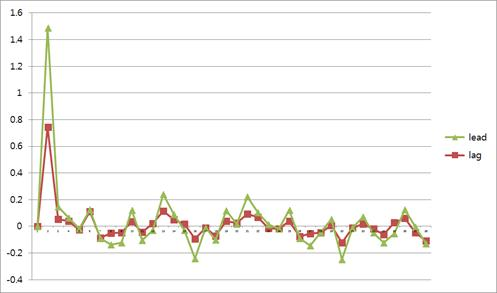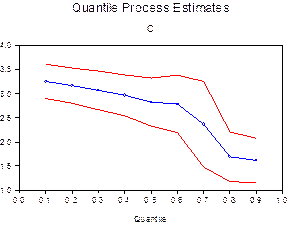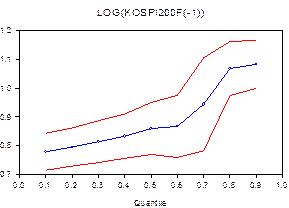Figure 4. Scatter Plot for Returns, Cross Correlogram and Quantile Regression for Log Levels.

Table 5. Test of Efficient Market Hypothesis.

 Dependent Variable: LOG(KOSPI) Variable Coefficient Std. Error t-Statistic Prob. C 2.553984 0.182763 13.97429 0.0000 LOG(KOSPI200F(-1)) 0.909635 0.032930 27.62334 0.0000 F-statistic 763.0489 Durbin-Watson stat 0.274058
 Null Hypothesis: C(1)=0, C(2)=1 Null Hypothesis Summary: Normalized Restriction (= 0) Value Std. Err. C(1) 2.553984 0.182763 -1 + C(2) -0.090365 0.032930 Restrictions are linear in coefficients.

In Econometrics, semiparametric estimation is important. It is based on fewer (distributional) assumptions, and estimators are devised from characteristics of population. (Greene, 2008)

Least absolute deviations (LAD) estimation is one example of semiparametric estimation. Ordinary east squares (OLS) estimator has shortcomings such as giving large weights on large deviation from the regression. LAD overcomes this defect by minimizing the sum of absolute value of the deviations from the regression. LAD estimator is a special case of the quantile regression. Koenker and Bassett (1978, 1982), Huber (1967) and Roger (1993) produced an estimator of asymptotic covariance matrix of the quantile regression estimator. LAD estimates the median regression. It is the solution to the quantile regression when q=0.5.

Prob(y < x’β) =q                                  (6-1)

Regression output and test result show that future market is inefficient in that it predicts the future prices (returns) to be incorrect systematically.

3.2. Cointegration Between Spot and Future Index

There is spurious regression problem in regression with nonstationary series. The test of cointegration is performed by equilibrium error. If this (that is, difference between two nonstationary series) is stationary, we can say that there is a long-run equilibrium relationship. From Fig 5., it seems there to exist cointegrating relationships between stock index spots and futures.

If the cointegrating rank of a system exceeds one, it is not possible to estimate behavioral relationships. Enders (1995) provides the estimation of money demand example.Figure 5. Efficient Market Hypothesis- Residuals From Spot – Future Regression .

The number of independent cointegrating vectors is called cointegrating rank. If the cointegrating rank of a system exceeds one, it is not possible to estimate behavioral relationships. Enders (1995) provides the estimation of money demand example. If the two series are both I (1), then residuals between them may be stationary (stable). (Engle and Granger Method, Hill et all., 2008)

Johansen(1988, 1991) and Stock and Watson(1988) based cointegration test on the VAR approach. The null hypothesis that there is r or fewer cointegrating vectors tested by TRACE test statistic:

-T Σ ln [1-(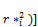(6-2)

We refer this statistic to the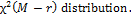Trace and maximum eigenvalue tests indicate no cointegration relationship between index variables at the 0.05 % level

Table 6. Cointegration Test.

 Unrestricted Cointegration Rank Test (Trace) Hypothesized Trace 0.05 No. of CE(s) Eigenvalue Statistic Critical Value Prob.** None 0.020218 4.822556 15.49471 0.8274 At most 1 0.000914 0.206551 3.841466 0.6495 Unrestricted Cointegration Rank Test (Maximum Eigenvalue) Hypothesized Max-Eigen 0.05 No. of CE(s) Eigenvalue Statistic Critical Value Prob.** None 0.020218 4.616006 14.26460 0.7893 At most 1 0.000914 0.206551 3.841466 0.6495 * denotes rejection of the hypothesis at the 0.05 level **MacKinnon-Haug-Michelis (1999) p-values

3.3. Distributed Lags Model

In macroeconomics, monetary shock affects output across several periods. So, the output at t,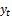, is determined by lagged variables of monetary stock.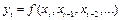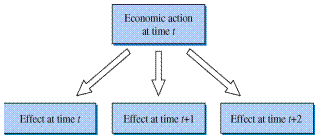Figure 6. Dynamic Models (Hill et al. 2008).

(3rd degree) Polynomial distributed lags model is as follows: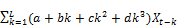(7)

In price expectation cases, Almond DL models are used for estimating delay and interim multipliers. This insists that lag weights lie on a straight line: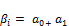i, i = 0,1,2,3,4

We can estimate the effects of lagged futures index on spot index through this method.

Table 7. Polynomial Distributed Lags Model(L=3).

 Time Lags Coeff Std Error T-Stat Signif Constant 2.886 0.237 12.164 0.000 LOGKOSPIF 0.568 0.071 8.033 0.000 LOGKOSPIF{1} 0.330 0.027 12.236 0.000 LOGKOSPIF{2} 0.156 0.023 6.916 0.000 LOGKOSPIF{5} -0.068 0.031 -2.202 0.029 LOGKOSPIF{6} -0.074 0.022 -3.383 0.001 LOGKOSPIF{7} -0.060 0.015 -3.930 0.000 Long-run(Interim) Multiplier 0.853

Table 8. Polynomial Distributed Lags Model(L=2).

 Time Lags Coeff Std Error T-Stat Signif Constant 3.322 0.267 12.427 0.000 LOGKOSPIF 0.114 0.012 9.508 0.000 LOGKOSPIF{1} 0.183 0.018 9.897 0.000 LOGKOSPIF{2} 0.212 0.020 10.474 0.000 LOGKOSPIF{3} 0.210 0.018 11.413 0.000 ………. Long-run(Interim) multiplier 0.771

3.4. Volatility and Efficiency

Fama(1991) surveys volatility tests for assessing market efficiency. He examined the tests with regard to whether the variation in expected return is rational. In this study, we consider only the existence of asymmetric volatility in view of the response of (efficient) market to the news.

ARCH(AutoRegressive Conditional Heteroscedasticity) treats error variances as affected lagged error terms. In general, bad and good news are assumed to affect asset returns symmetrically.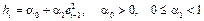(8)

Generalized ARCH(GARCH) treats bad and good "news"

Symmetrically. (Fig 7, Top Panel, Generalized standard deviation)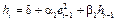But, there may have asymmetrical effects on volatility. When negative news hits stock market, equity prices show a turbulent phase. Threshold(T-) GARCH allows asymmetric effects on asset price volatility for bad and good news. Estimation results show the existence of asymmetries due to bad news. (Fig. 7, Middle Panel, TGARCH11) This implies that there is asymmetry in which market incorporates outside information(news).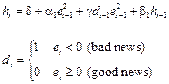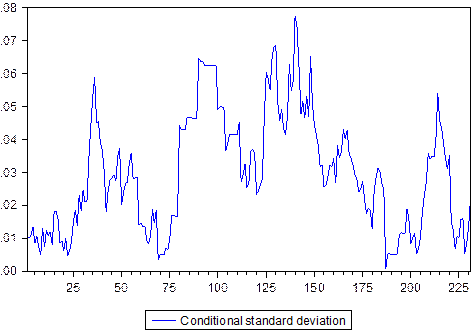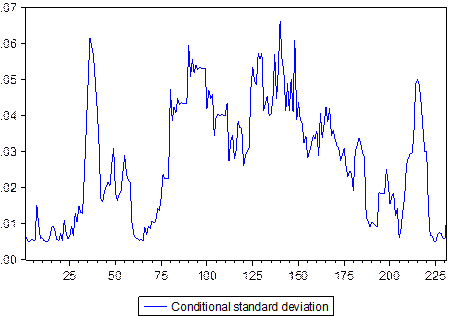Figure 7. Generalized ARCH Models.

GARCH-in-mean (-M) model suggests adding time-varying intercept to variance equation. (GARCHM)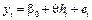Finally, negative answers in empirical conclusion for efficiency could be justified by these facts. Implicit risk-neutrality assumption has some problems. If investors are risk-averse, future index contains time-varying risk premium. By using GARCH-in-Mean model, we can get evidence of premium. (Fig. 7, Bottom Panel, Conditional standard deviation)

Finally, in contrast to Bae et al. (2004), the level of stock index futures reduces the spot market volatility. This can be seen in estimation result of GARCH variance equation.

Table 9. Generalized ARCH Models.

 Dependent Variable: LOG(KOSPI200F) GARCH = C(3) + C(4)*RESID(-1)^2 + C(5)*GARCH(-1) Variable Coefficiant Std. Error z-Statistic Prob. GARCH -6.204555 2.528911 -2.453450 0.0141 C 5.571066 0.001032 5398.612 0.0000
 Dependent Variable: DLOG(KOSPI) GARCH = C(1) + C(2)*RESID(-1)^2 + C(3)*GARCH(-1) + C(4) *LOG(KOSPI200F) Variable Coefficient Std. Error z-Statistic Prob. Variance Equation C 0.000325 0.000143 2.274447 0.0229 RESID(-1)^2 -0.104018 0.017071 -6.093387 0.0000 GARCH(-1) 0.769961 0.116240 6.623922 0.0000 LOG(KOSPI200F) -5.62E-05 2.54E-05 -2.215384 0.0267

3.5. Vector Autoregression

Lag lengths in a VAR is determined by log-likelihood, likelihood ratio or information criteria. Most criteria show that optimal lag length is three.

An (reduced-form) VAR can be expressed more easily in matrix form as:

Y t =CY t-1 +V t

The real dynamics of impulse responses is complicated by: we should identify the correct shock from unobservable data.(Hill et al., 2008) This complication leads to the identification problem.

More precisely, a structural form is expressed as:

BY t =AY t-1 +E t10                                      (9)

where Yt =(St, Ft)'.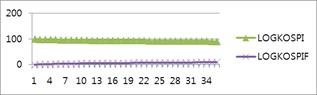Figure 8. Variance Decomposition of Forecast Error for Spot Index.

Forecast error variance decomposition reveals relatively large explanatory power of future index for the forecast of spot index compared to vice versa.(Figure 8 and 9)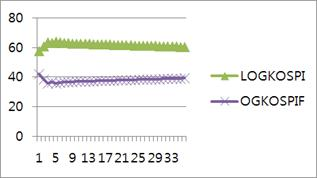Figure 9. Variance Decomposition of Forecast Error for Future Index.

We can also derive impulse responses to a standard deviation shock. From both the responses in returns and in levels, we can find the larger effects in spot rate to future rate.11 This indirectly supports market efficiency in future index market.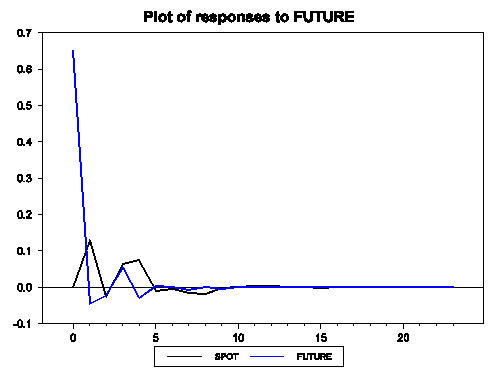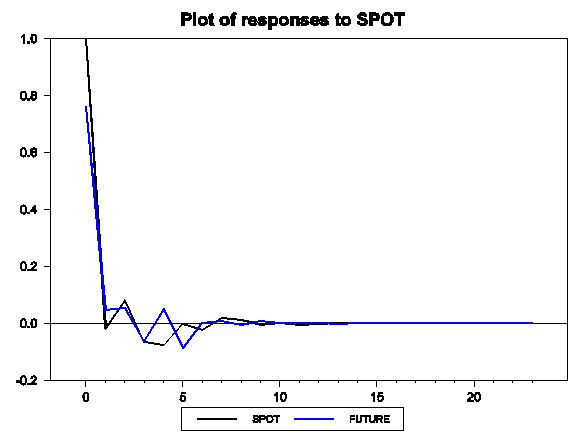Figure 10. Impulse Response in Contemporaneous Returns.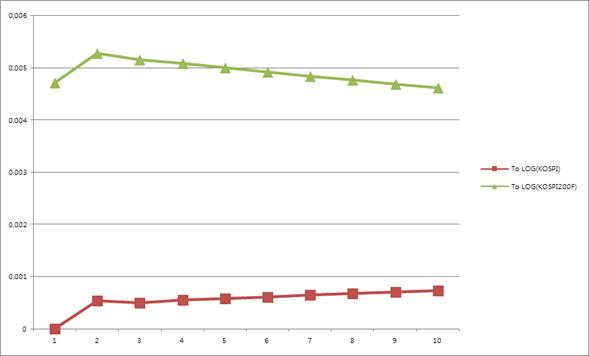Figure 11. Impulse Response in Contemporaneous Log Levels.Figure 12. Impulse Response in Lagged Returns.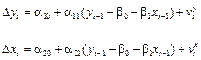(x: future, y: spot)

In addition, estimation results for error correction model reveals short-run adjustment process (market efficiency) for stock index futures contract is valid, but not for spots contract.

3.6. Discussion

It is notable that previous literatures provide the results those futures (eg. foreign exchange) market is inefficient, contrasted to this study. In this section, we compare the forecasting performances each other using a few forecast models. We compared forecast performances for future spot index among AR, DL, ARMA, ARDL models. We used RMSE, MAE, and Theil coefficient. From all the measures of forecast performances, we find the fact that lagged future index is best predictor for spot index.

Finally, the hypothesis of unbiasedness of future index is based on the assumption that rational expectation and risk-neutrality.

Table 10. Forecasting Performance, Omitted Variable, and Specification Test (J-Test).

 Random Walk Lagged Future ARMA ARDL RMSE 72.5 32 71.9 67 MAE 61 25 60 55
 Ramsey RESET Test Specification: LOG(KOSPI) C LOG(KOSPI200F(-1)) LOG(KOSPI(-1)) Omitted Variables: Squares of fitted values Value df Probability t-statistic 0.862282 226 0.3894 F-statistic 0.743531 (1, 226) 0.3894 Likelihood ratio 0.755449 1 0.3848 F-test summary: Sum of Sq. df Mean Squares Test SSR 2.90E-05 1 2.90E-05 Restricted SSR 0.008833 227 3.89E-05 Unrestricted SSR 0.008804 226 3.90E-05 LR test summary: Value df Restricted LogL 842.8930 227 Unrestricted LogL 843.2708 226
 Dependent Variable: LOG(KOSPI) Variable Coefficient Std. Error t-Statistic Prob. C 2.548679 0.005422 470.0335 0.0000 LOG(KOSPI200F(-1)) 0.913880 0.001894 482.5711 0.0000 LOG(KOSPI(-1)) -0.002388 0.002016 -1.184352 0.2375 RESID03 1.002042 0.001879 533.3088 0.0000
 Dependent Variable: LOG(KOSPI) Variable Coefficient Std. Error t-Statistic Prob. C 2.533351 0.169013 14.98906 0.0000 LOG(KOSPI200F(-1)) 0.843878 0.146625 5.755355 0.0000 LOG(KOSPI200F(-2)) 0.069495 0.146876 0.473156 0.6366 RESID02 1.009753 0.154236 6.546805 0.0000

Recent behavioral finance school caused the efficient market hypothesis to be rejected among the economists. Most important topic in this area is inefficient market hypothesis.

First, there is equity-premium puzzle. This incorporates the behavioral pattern by which investors mind selling low-priced equities. This is kind of endowment effect. Second, there are representative heuristics in investor’s behavior. Investors misunderstand the luck in investment as the equity having good fundamental. Third, there is over-reaction in investment patterns. This is sometimes called "law of small numbers".

All these hypotheses explain parts of rejection of efficient market hypothesis.

4. Summary and Limitations

We found the fact that we cannot predict spot stock index by its own index, but can predict fairly well by futures index. That is, spot index market is efficient, but future market is inefficient. This is the main questions of efficient market or random walk hypothesis in financial econometrics.

The earliest model of asset prices is the martingale model. This contains the notion of fair game which could be found in contingent commodity model.

In this paper, we test the argument by a few econometric approach developed recently. Market-efficiency debates using statistical models like variance bounds, Euler equations, CAPM and APT may be applied more profitably to the measurement of efficiency rather than hypothesis tests. (Campbell et al. 1997)

According to test and estimation results, there are (significant) level relationships between stock index Futures and Spots. We can find, though, short-run efficiency (error correction adjustment) is only valid. Spot market became almost efficient through its own lagged effects in Korea.

References

1. Antoniou A. and P. Holmes (1996), "Futures Market Efficiency: The Unbiasedness Hypothesis and Variance-Bounds Tests: The Case of FTSE-100 Futures Contract", Bulletin of Economic Research, 48, 2: 0307-3378.
2. Bae, Kwon and Park (2004), "Futures Trading, Spot Market Volatility, and Market Efficiency: The Case of Korean Index Futures Markets", Journal of Futures Markets, 24, 12: 1195-1228.
3. Blanchard O. and S. Fischer(1989), Lectures on Macroeconomics, The MIT Press.
4. Campbell J. and Mankiw, N.G.(1987), "Permanent and Transitory Components in Macroeconomic Fluctuations ", American Economic Review Proceedings.
5. Campbell J.(1997), The Econometrics of Financial Markets, Princeton Univ. Press: Princeton NJ.
6. Cogley T.(1990), "International Evidence on the Size of the Random Walk in Output ", Journal of Political Economy 98(3).
7. Engle,R.F.(1982) "Autoregressive conditional heteroscedasticity with estimates of the variance of United Kingdom inflation", Econometrica 50: 987–1007.
8. Fama, E.F.(1970) "Efficient capital markets: a review of theory and empirical work" Journal of Finance 25: 383–417.
9. Fama, E.F.(1991) Market Efficiency, Long-term Returns, and Behavioral Finance, Journal of Financial Economics 49: 283–306.
10. Hill C, W. Griffiths and G. Lim(2008) Principles of Econometrics, 3rd Ed.Wiley.
11. Levich (2001)"Empirical Studies of Exchange Rates: Price Behavior, Rate Determination and the Market Efficiency", Handbook of International Economics, Elsevier Publisher.
12. Lim K-P. and R. Brooks(2011) "The Evolution of Stock Market Efficiency Over Time: A Survey of The Empirical Literature", Journal of Economic Surveys, Volume 25, Issue 1, February:69–10.
13. Nelson C. and Plosser C.(1982), "Trends and Random Walks in Macroeconomic
14. Time Series ", Journal of Monetary Economics 10.
15. Pesaran H, Y. Shin and R. Smith(2001), "Bound Testing Approaches to the Analysis of Level Relationships ", Journal of Applied Econometrics 16: 289-326.
16. Pesaran H, Y. Shin(2000), "Structural Analysis of Vector Error Correction Models with Exogenous I(1) Variable", Journal of Econometrics 97: 293-343.
17. Pesaran H, and Y. Shin(1999), "An Autoregressive Distributed Lag Modelling Approach to Cointegration Analysis ", Econometrics and Economic Theory in the 20th Century:, Cambridge Univ. Press.
18. Romer D.(2006) Advanced Macroeconomics, International Edition.
19. Yen, G. and Lee, C.F.(2008) "Efficient market hypothesis (EMH): past, present and future", Review of Pacific Basin Financial Markets and Policies 11: 305–329.

 This study was performed under the assistance of 2015 KNUT Academic Program.

 They argue that for testing, it is necessary to construct equilibrium equation, which is criticized as joint-hypothesis or bad model problem.(Jarrow and Larsson, 2012)

 It is important to distinguish the information set that is considered among: Weak-form efficiency(only the history of prices themselves), Semistrong-form(publicly available information), and Strong-form(private information).

 We use comparatively new testing procedures in econometrics. They are quantile regression method of Roger(1993). The main advantage of this method is that it overcomes the defects of OLS estimator that it is too sensitive to outliers.

 In econometric version, RW1 may be the independently and identically distributed increments case. RW2 be independent increments, and RW3 uncorrelated increments, respectively.

 Campbell et al.(1997)

 Drift(expected rate change) are added to AR(1) estimation equation.

 Hereafter, if coefficient estimate is significant at 5%, 10%, we denote * and ** respectively.

 Campbell, Lo and MacKinlay (1997) for reviews.

 We impose long-run restriction so that the shocks in Future index has no effect on Spot index in the long-run.

 But, we should note the fact that if lagged future index is used, relative explanatory power in spot index is larger.

 Contents 1. 2. 2.1. 2.2. 2.3. 3. 3.1. 3.2. 3.3. 3.4. 3.5. 3.6. 4.
Article ToolsAbstractPDF(502K)